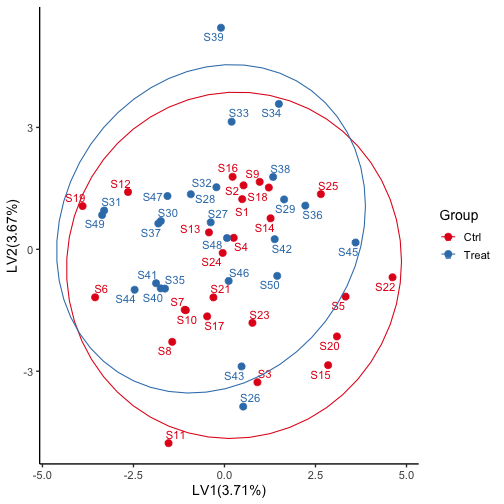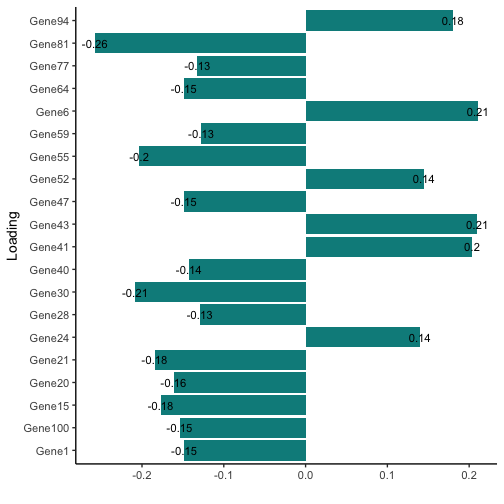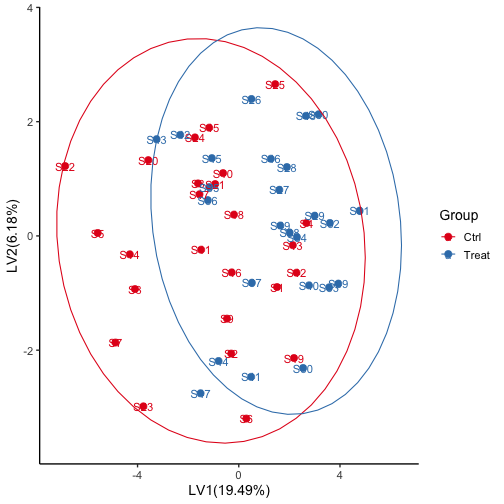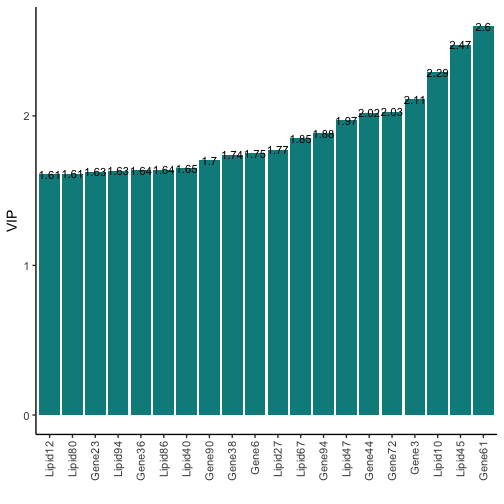# o2plsda: Omics data integration with o2plsda

## Description

o2plsda provides functions to do O2PLS-DA analysis for multiple omics integration.The algorithm came from “O2-PLS, a two-block (X±Y) latent variable regression (LVR) method with an integral OSC filter” which published by Johan Trygg and Svante Wold at 2003. O2PLS is a bidirectional multivariate regression method that aims to separate the covariance between two data sets (it was recently extended to multiple data sets) (Löfstedt and Trygg, 2011; Löfstedt et al., 2012) from the systematic sources of variance being specific for each data set separately. It decomposes the variation of two datasets in three parts:

• a joint part that is correlated (predictive) to $$X$$ and $$Y$$ (i.e. variation related to both $$X$$ and $$Y$$), denoted as $$X/Y$$ joint variation in $$X$$ and $$Y$$: $$TW^\top$$ and $$UC^\top$$,
• a part contained inside $$X/Y$$ that is uncorrelated (orthogonal) to $$X$$ and $$Y$$: $$T_{yosc} W_{yosc} ^\top$$ and $$U_{xosc} C_{xosc}^\top$$,
• A noise part for $$X$$ and $$Y$$: $$E_{xy}$$ and $$F_{yx}$$.

The number of columns in $$T$$, $$U$$, $$W$$ and $$C$$ are denoted by as $$nc$$ and are referred to as the number of joint components. The number of columns in $$T_{yosc}$$ and $$W_{yosc}$$ are denoted by as $$nx$$ and are referred to as the number of $$X$$-specific components. Analoguous for $$Y$$, where we use $$ny$$ to denote the number of $$Y$$-specific components. The relation between $$T$$ and $$U$$ makes the joint part the joint part: $$U = TB_U + H$$ or $$U = TB_T'+ H'$$. The number of components $$(nc, nx, ny)$$ are chosen beforehand (e.g. with Cross-Validation).

### Cross-Validation

In order to avoid overfitting of the model, the optimal number of latent variables for each model structure was estimated using group-balanced Monte Carlo cross-validation (MCCV). The package could use the group information when we select the best parameters with cross-validation. In cross-validation (CV) one minimizes a certain measure of error over some parameters that should be determined a priori. Here, we have three parameters: $$(nc, nx, ny)$$. A popular measure is the prediction error $$||Y - \hat{Y}||$$, where $$\hat{Y}$$ is a prediction of $$Y$$. In our case the O2PLS method is symmetric in $$X$$ and $$Y$$, so we minimize the sum of the prediction errors: $$||X - \hat{X}||+||Y - \hat{Y}||$$.

And we also calculate the the average $$Q^2$$ values:

$$Q^2$$ = 1 - $$err$$ / $$Var_{total}$$;

$$err$$ = $$Var_{expected}$$ - $$Var_{estimated}$$;

Here $$nc$$ should be a positive integer, and $$nx$$ and $$ny$$ should be non-negative. The 'best' integers are then the minimizers of the prediction error.

The O2PLS-DA analysis was performed as described by Bylesjö et al. (2007); briefly, the O2PLS predictive variation [$$TW^\top$$, $$UC^\top$$] was used for a subsequent O2PLS-DA analysis. The Variable Importance in the Projection (VIP) value was calculated as a weighted sum of the squared correlations between the OPLS-DA components and the original variable.

## Installation

install.package("o2plsda")


## Examples

library(o2plsda)

##
## Attaching package: 'o2plsda'

## The following object is masked from 'package:stats':
##

set.seed(123)
# sample * values
X = matrix(rnorm(5000),50,100)
# sample * values
Y = matrix(rnorm(5000),50,100)
rownames(X) <- paste("S",1:50,sep="")
rownames(Y) <- paste("S",1:50,sep="")
## gene names
colnames(X) <- paste("Gene",1:100,sep="")
colnames(Y) <- paste("Lipid",1:100,sep="")
##scaled
X = scale(X, scale = TRUE)
Y = scale(Y, scale = TRUE)
## group factor could be omitted if you don't have any group
group <- rep(c("Ctrl","Treat"), each = 25)


Do cross validation with group information

set.seed(123)
## nr_folds : cross validation k-fold (suggest 10)
## ncores : parallel paramaters for large datasets
cv <- o2cv(X,Y,1:5,1:3,1:3, group = group, nr_folds = 10)

## #####################################

## The best parameters are nc = NA, nx = NA, ny = NA

## #####################################

## The Qxy is NA and the RMSE is: NA

## #####################################

#####################################
# The best parameters are nc =  5 , nx =  3 , ny =  3
#####################################
# The Qxy is  0.08222935  and the RMSE is:  2.030108
#####################################


Then we can do the O2PLS analysis with nc = 5, nx = 3, ny =3. You can also select the best parameters by looking at the cross validation results.

fit <- o2pls(X,Y,5,3,3)
summary(fit)

##
## ######### Summary of the O2PLS results #########
## ### Call o2pls(X, Y, nc= 5 , nx= 3 , ny= 3 ) ###
## ### Total variation
## ### X: 4900 ; Y: 4900  ###
## ### Total modeled variation ### X: 0.286 ; Y: 0.304  ###
## ### Joint, Orthogonal, Noise (proportions) ###
##                X     Y
## Joint      0.176 0.192
## Orthogonal 0.110 0.112
## Noise      0.714 0.696
## ### Variation in X joint part predicted by Y Joint part: 0.906
## ### Variation in Y joint part predicted by X Joint part: 0.908
## ### Variation in each Latent Variable (LV) in Joint part:
##     LV1   LV2   LV3   LV4   LV5
## X 0.037 0.037 0.039 0.031 0.032
## Y 0.047 0.042 0.036 0.035 0.032
## ### Variation in each Latent Variable (LV) in X Orthogonal part:
##     LV1   LV2   LV3
## X 0.047 0.034 0.029
## ### Variation in each Latent Variable (LV) in Y Orthogonal part:
##     LV1   LV2   LV3
## Y 0.046 0.034 0.032
##
## ############################################

######### Summary of the O2PLS results #########
### Call o2pls(X, Y, nc= 5 , nx= 3 , ny= 3 ) ###
### Total variation
### X: 4900 ; Y: 4900  ###
### Total modeled variation ### X: 0.286 ; Y: 0.304  ###
### Joint, Orthogonal, Noise (proportions) ###
#               X     Y
#Joint      0.176 0.192
#Orthogonal 0.110 0.112
#Noise      0.714 0.696
### Variation in X joint part predicted by Y Joint part: 0.906
### Variation in Y joint part predicted by X Joint part: 0.908
### Variation in each Latent Variable (LV) in Joint part:
#      LV1     LV2     LV3     LV4     LV5
#X 181.764 179.595 191.210 152.174 157.819
#Y 229.308 204.829 175.926 173.382 155.934
### Variation in each Latent Variable (LV) in X Orthogonal part:
#      LV1     LV2     LV3
#X 227.856 166.718 143.602
### Variation in each Latent Variable (LV) in Y Orthogonal part:
#      LV1     LV2     LV3
#Y 225.833 166.231 157.976


Extract the loadings and scores from the fit results and generated figures

Xl <- loadings(fit,loading="Xjoint")
Xs <- scores(fit,score="Xjoint")
plot(fit,type="score",var="Xjoint", group=group)plot(fit,type="loading",var="Xjoint", group=group,repel=F,rotation=TRUE)Do the OPLSDA based on the O2PLS results and calculate the VIP values

res <- oplsda(fit,group, nc=5)
plot(res,type="score", group=group)vip <- vip(res)
plot(res,type="vip", group = group, repel = FALSE,order=TRUE)